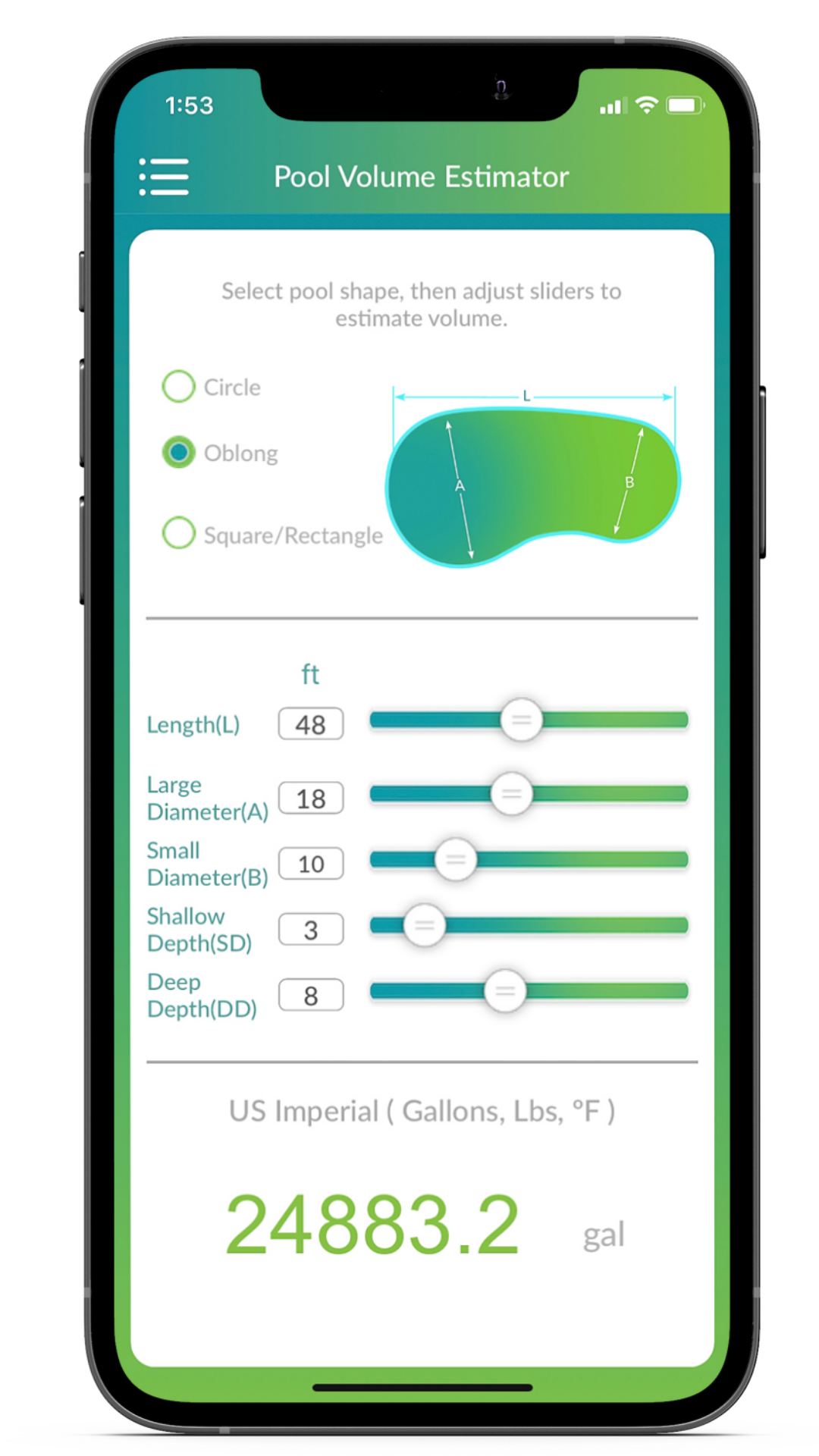1. Help Center
2. Orenda App
3. How to use the Orenda App

# How to use the Pool Volume Estimator

## The Pool Volume Estimator in the Orenda App uses dimensions to estimate your pool volume.

Our pool volume estimator allows you to select three common shapes of pools and spas:

• Circle
• Oblong (kidney)
• Square/rectangle

Depending on the shape of your pool, the section below will show the dimensions needed for a volume estimate.

The estimator works by calculating cubic area of your pool, and multiplying that by a factor to convert that cubic area into gallons. Specifically (cubic feet x 7.48 = gallons).

### Step-by-step instructions

1. Select the general shape of your pool (circle, oblong, square/rectangle). The parameters below will change according to what you select.
2. Either tap the number and type in the dimension (typing allows for decimals), or slide the dial to adjust the dimensions accordingly.
3. In real-time, the estimate at the bottom of the screen will show you approximately how many gallons (or liters, if using the metric system in App Settings) the pool is.### How accurate is the Pool Volume Estimator?

Our estimator is only as accurate as the dimensions you input. Unless the pool is pre-fabricated and the volume is absolutely known by the manufacturer, no pool estimate will be 100% accurate, especially with plumbing underground of unknown lengths.

Do the best you can with the dimensions you measure, and the estimator will accurately use the formula of

Cubic feet x 7.48 = gallons

The only way to know your pool's volume exactly right is to use water meters when initially filling the pool. Water meters will tell you exactly how much water it took to fill the pool, and it includes the plumbing.